#2017 iT 邦幫忙鐵人賽
DAY 8
1
Big Data

## Day08 R語言的函式(Function)

Write once, run anywhere "at R"

## R語言函式:

``````function(參數1,參數2)
{
return(回傳參數)
}
``````

*空括號表示函式不需要任何參數。

R語言內建的函式非常完整，從一般的數學算數、三角函數、排序、字串、日期及統計函式都有支援，再加上安裝Package後新增的函式，如果我們能熟悉且善用，在R語言中真正寫起程式的機會很少，我們就能將時間專注在使用上。## 內建算數函式

• seq : Sequence Generation 連續數產生(也可以設定起始結束及間隔值參數)
• mean: Arithmetic Mean 算數平均
• sum: Sum of Vector Elements 向量加總

``````#Create a sequence of numbers from 1 to 10.
print(seq(1, 10))

#Find mean of numbers from 1 to 100.
print(mean(1:100))

#Find sum of numbers frm 1 to 100.
print(sum(1:100))
``````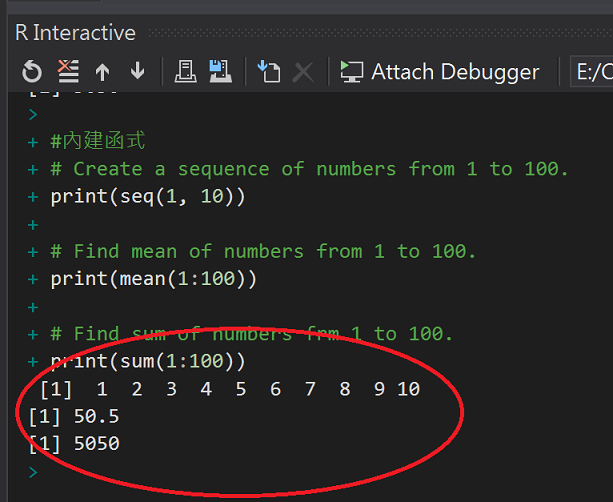## 自建函式

``````#Create a function to get Odd count
OddCount.function <- function(x) {
o <- 0
for (n in x) {
if (n %% 2 == 1) {
o <- o + 1
}
}
return(o)
}

#Call the function
OddCount.function(c(1,2,3,4,5))
``````## 帶有預設值的自建函式

• 如果有輸入參數，依照輸入的參數組相乘，並回傳乘積
• 如果沒有輸入參數，依照預設參數組7、9，回傳乘積63

``````#自建函式(有預設值)
#Create a function with arguments.
multiply.function <- function(x = 7, y = 9) {
result <- x * y
print(result)
}

#Call the function without giving any argument.
multiply.function()

#Call the function with giving new values of the argument.
multiply.function(6, 8)
``````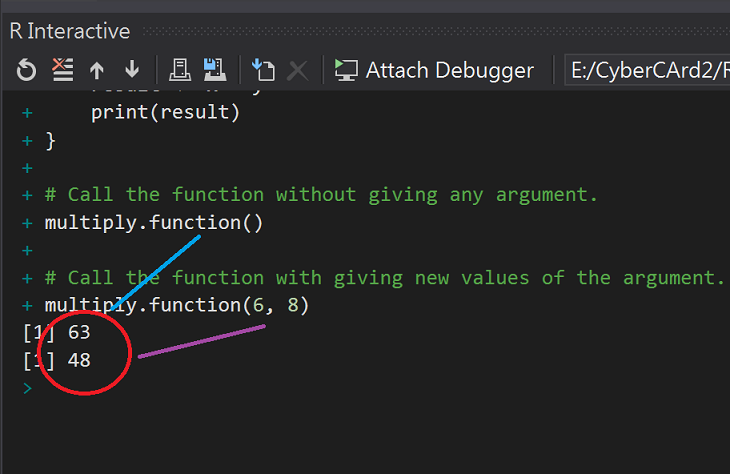41J、記者正確!

## Call by value and Call by reference

R語言的函式通常是傳值呼叫

### call by value

``````add.fucntion <- function(x) {
x <- x + 1
x
}
x <- 1
x
``````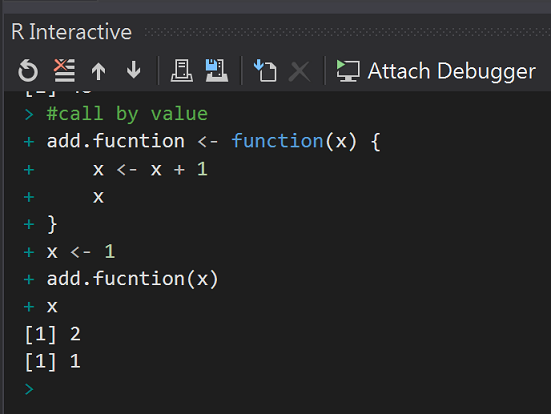### call by reference

``````add.fucntion <- function(x) {
value <- x + 1
eval.parent(substitute(x <- value))
}
x <- 1
x
#help(eval.parent) Evaluate an (Unevaluated) Expression
#help(substitute) Substituting and Quoting Expressions
``````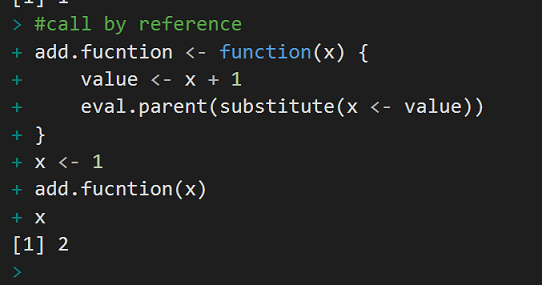R語言沒有宣告變數的概念，使用變數前不需要事先宣告型別也!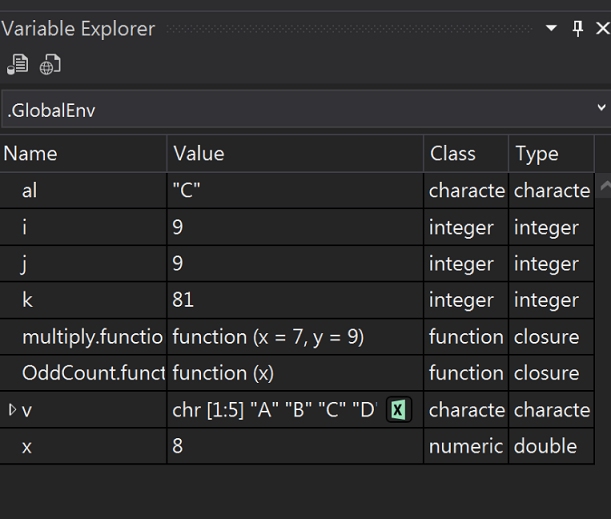``````rm(list = ls())
``````A Big Function:Emirates A3802015-10 攝於巴塞隆納機場,加泰隆尼亞(spain)

R語言與機器學習見面會30